International Journal of Energy and Power Engineering
Volume 4, Issue 5, October 2015, Pages: 280-286

Monthly Stream Flow Predition in Pungwe River for Small Hydropower Plant Using Wavelet Method

Miguel Meque Uamusse1, 3, *, Petro Ndalila2, Alberto JúlioTsamba3, Frede de Oliveira Carvalho4, Kenneth Person1

1Department of Water Resource,Lund University, Lund, Sweden

2Department of Mechanical Engineering, Mbeya University of Science and Technology, Mbea, Tanzania

4Departamento de Engenharia Química, Universidade Federal de Alagoas, Brazil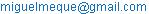(M. M. Uamusse)

Miguel Meque Uamusse, Petro Ndalila, Alberto JúlioTsamba, Frede de Oliveira Carvalho, Kenneth Person. Monthly Stream Flow Predition in Pungwe River for Small Hydropower Plant Using Wavelet Method.International Journal of Energy and Power Engineering.Vol.4, No. 5, 2015, pp. 280-286. doi: 10.11648/j.ijepe.20150405.17

Abstract: This study investigates the effect of discrete wavelet transform data pre-processing method on neural network-based on monthly streamflow prediction models to produce energy from small Hydro power plant in along of Pungwe river basin in Mozambique. The study used data from Vanduzi gauging a station which is along of Pungwe river basin. Eight different single-step-ahead neural monthly stream flow prediction models were developed. Coupled simulation between MATLAB and wavelet neural network was used to solve the problem. Different models were teste with the same sample and found that, Artificial Neural Network had the best performance. The main objective of this research project is to analyze the monthly stream flow prediction in the Pungwe river, so as to make suitable decisions in dry or wet spells also to resolve probable conflicts about water recourses.

Keywords: Renewable Energy, Hydropower, Wavelet Artificial Neural Network, Monthly Flow Prediction

1. Introduction

According currently energy crisis, energy consumptions is become a big problem in Mozambique especially the big and concentrated cities such Maputo, Beira and Nampula. For the reason is to bring renewable energy hydropower technology to replace current energy mix in Mozambique. One of the best energy alternative in Mozambique is the conversion of Hydropower energy because the source is abundant, is clean energy, is renewable, and it can creat more job opportunity (EDM, 2015; IEA, 2009; Cuamba et al.,2010; Uamusse et al.,2014 and FUNAE).

To study about level of river flow and its fluctuations time at different times of the year is one of the significant factor to achieve sustainable development for water resource issues and energy planning Hydropower energy is one of promising clean energy technologies, however this technology has some challenges compare with biomass, solar, and wind energy. The most challenging issue here is high capital investment cost.

Steam flow perdition is essential in all activities involving the operation and optimization of water resources management and planning issues, than for this reason, the development of Mathematical model to be able provide more reliable long-term forecasting has attracted the attention of hydrologist throuth time (Santos and Silva,2013; Seo et al,. 2014).

In this article, two-hybrid models are studied, Wavelet Neural Fuzzy and Wavelet Artificial Neural Network (WANN) for flow prediction of the Pungwe River. Water flow prediction can play an important role in water resources management over river basin, in this work different methods have been use to predict Monthly flow. The main objective of this research project is to analyze the monthly stream flow prediction in the Pungwe river, so as to make suitable decisions in dry or wet spells also to resolve probable conflicts about water recourses.

Nowadays many studies of rivers flows and aplications of artificial networks to varios aspects of hydrological modelling has ben constituted interesting to some scientists( Nourani et al., 2013; Santos and Silva 2013;Nayak  et al.,2013, Muhanmadi et al., 2008; Sreekath et al 2009; Partal and Kisi, 2007 and Seo et al., 2014). The interest of this Hydrological modeling is to improve performance of artificial neural networks (ANN) to predict seasonal time series. Several structures of ANN presented to predict seasonal time series.

Several scientific paper of Hydrology about streamflow prediction methods, time series analysis have been demonstrated the good performance of WANN, ARIMA and WANFIS models and inclusion richer information and good systematic way to modeling flow( Santos and Silva 2014; Rezaeianzadeh et all.,2014; Nejad and Nourani 2012; Kisi, 2007 and Kisi, 2008).

Nonetheless to use wavelet transforms for time series treatment and ANN although flexibility and applicabiliy are still present difficulties (Neurani et all 2013, Santos and Silva, 2013). The application of some methods to pre-process imput data has been highlighted as an efficient alternative to improve the performance of ANN models like wavelet which has recently received attentions because provide useful decomposition os original time series into high to low frequence components.

The knowledge of stream flow system along the Pungwe River will help to plan how many Hydro power  plants can be built along the river as well as other consumption such as; domestic, animal, and irrigation, so as avoid possible conflicts of sharing water along the river basin. Monthly modeling and prediction of Pungwe river flow  was used by wavelet neural network (WNN) method in combination with neural networks (NN) and Neural Fuzzy Model using monthly flow data from one gauging stations  in Pungwe river basin in Mozambique. Other Motivation of this research investigation is Renewable energy has been considered as one of the key elements to address climate change issues  due to global warming and because the fossil fuels contribute to increase greenhouse gases (Kisi,2006, Kisi 2008, Nourani et al,. 2014; Naghizadeh et al,. 2012).

2. Description of the Study Area

The Pungwe river basin is located between latitudes 18S and 20S and the longitudes 33E and 35E and covers an total catchment area of some 31150 km2 according to the new estimate, with a perimeter of 318.08 km. The Pungwe river is born in eastern highlands in Zimbabwe and flows eastwards through the Mozambican provinces of Manica and Sofala on its way to the Indian Ocean at Beira. The Zimbabwean part of the basin is 1 460.7 km2 and the Mozambican part is 29 689.8 km2.

The climate of Pungwe River basin stretches over two climate types, the is tropical of 6 months dry season and the remaining 6 months is rainy season annually. The average annual precipitation is 2000 mm the months of December to February with an average monthly rainfall of 300 mm in the month of February. In the mountains as shown in Figure 1 there is normally rain every month of the year, with a concentration in November-April, while the area east of the mountains has a pronounced concentration of the rain for the warm season November-April, and mainly no precipitation at all from May to October. In the eastern region, near Beira, the climate is classified as tropical humid, with a temperature variation from 22° in July to 29°C in January. The mean rainfall varies from 300 mm in January to 20 mm in July.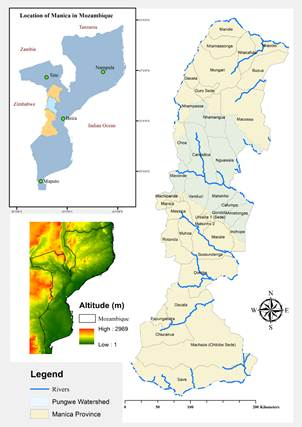Figure 1. Location of Pungwe river basin in Manica, Mozambique.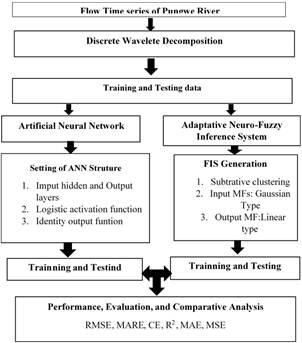Figure 2. Flowchart of methodolo.

3. Methodology

For the purpose of this research and to achieve the objectives the following activities we carried out Literature :(Santos and Silva, 2014; Khan, 2012; Nourani et al., 2013; Santos and Silva 2013; Nayak et al.,2014; Muhanmadi et al.,2010; Sreekath et al,. 2009; Partal and Kisi 2007, Seo et al., 2014), materials, Matlab technology and data collection for 588 month and 49 years.

The Figure 2 its shows a flowchart for flow predition using artificial neural networw and adaptive neuro-fuzzy interference system.

3.1. Wavelet Analysis and Neural Network Model

The Wavelet transformation is a mathematical technique that transforms a signal in the time domain to frequency domain and by integral calculation, this transformation is described by equation 1. Wavelet neural network, a time series decomposes into the higher and low frequency components, multiple levels of details, sub-time series, which provide an interpretation of the original time series structure and history in both the time and frequency domains using a few coefficients (Badrzadeh, 2013; Alizdeh et al,. 2015, Nayaka et al,. 2013 and Solgi et al,. 2014, Chiu,1994).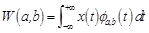(1)(2)

Where x (t) represents the temporal signal,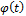is a mother wavelet function, in time and frequency domain and 𝑎 is scale parameter; 𝑏 is a position parameter and W (a, b) is the wavelet coefficients.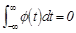(3)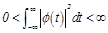Wavelet transformation is more effective instrumental than the Fourier transform during the studying non-stationary time series. The main advantage of wavelet transformation is the ability to simultaneously obtain information on the location and frequency of a signal, while Fourier Transformations its separates a time series in to sine waves of various frequencies. There are two types of wavelet transform where are Continuous wavelet Transform (CWT) and discrete wavelet transform (DWT).

The CWT calculations requires a significant amount of computation period and resoiurce. Considering the discrete nature of observed data of flow in the time series. DWT the original signal time series, passes through two complementary filter and emerges as one approximation to detail components, The DWT is most preferred in hydrological prediction( Kim and valdes,2003, Neurani et al., 2011; Honey et all, 2013; Dibike and solomatie,2001; kisi,2006)

In practical applications, hydrology researchers have access to a discrete time signal rather than continuous time signal (Santos and Silva 2014, Khan 2012, Nourani et al., 2013).

The same authors advise for the purpose of prognosis hybrid model ANN and ANFIS  following the scheme represented in the figure below. The Figure 3 show the schematic diagram of hybrid wavelet neural network model with one input and the time series signal was decomposed by wavelet transform and this sub signal as input into neural network.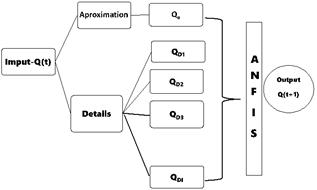Figure 3. Schematic diagram of hybrid WANN model.

In terms of development strategies of objective of this work, one question options presented is about kind of wavelet transform used (CWT,DWT) and combination with the ANNN and ANFIS. The table 1. summarizes the possibilities of the building models.

Table 1. Wavelet transform strategy used.

 Model Struture Wavelet transform Model ANFIS Model 1- NN Without Pré-Treatment Neural Network Model 2-ANFIS Without Pré-Treatment Neural-Fuzzy Model 3-CWTNN CWT Neurais Network Model 4-DWTNN DWT Neural Network Model 5-CWTANFIS CWT Neural-Fuzzy Model 6-DWTANFIS DWT Neural-Fuzzy

3.2. Artificial Neural Network (ANN)

Artificial neural network are computational and mathematical model with a wide ranges of applications have great ability in forecasting modeling for nonlinear hydrological time series (flow, and precipitation).

The ANN Procedure through the combination of neural networks to prediction components of frequency up to 5 layers, subsequently combining the simulated network values to reconstruction of the original signal by wavelet technique reconstruction, This Model is basically works with inverse decomposition process as shown in figure 5. For Artificial neural networks, the backpropagation algorithm automatically acquires the knowledge, but the learning process is relatively slow and analysis of the trained network is difficult (Jain et. al., 1999; Badrzadeh et al., 2013).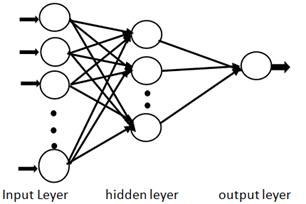Figure 5. Artificial Neural network Model Arquiteture.

3.3. Wavelet Artificial Neuro Fuzzy Inference System( WANFIS)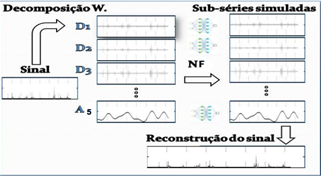Figure 4. Neuro fuzzy Model Arquiteture.

The WANFIS model was develop by using the wavelet sub series as ANFIS input, information of input and output data is convert into linguistically interpretable. In This Model, the Procedure is the same way like Artificial Neuro Network prediction components up to five layers of frequency, than combining the simulated network values for the reconstruction of the original signal using wavelet technical as shown in figure 4. Fuzzy logic systems, which can reason with imprecise information, are good at explaining their decisions but they cannot automatically acquire the rules they use to make those decisions For example, while neural networks are good at recognizing patterns, they are not good at explaining how they reach their decisions (Jang, 1993; Chiu, 1994; Neurani et al., 2011).

3.4. The Model Performance

The performance of different forecasting models was accessed in terms of goodness to fit once each of the model structures is calibrated using the training, validation data set and testing data. Degree of correlation was measure (R2) in equation 6. The equation 4 is coefficient of correlation(CE) was used to compare the goodness to fit between the measured flow and the simulated flow, mean-squared error (MSE) in equation 5 were used to evaluates the variance of error ( Nourani et al., 2013; Santos and Silva 2013; Nayak et al., 2014, Muhanmadi et al., 2010;Sreekath et al 2009, Partal and Kisi 2007 ). To calculate the root-mean-square error (RMSE), mean absolute error (MAE) and mean absolute relative error (MARE) will use remaining equations in the same literature.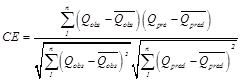(4)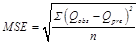(5)

EFF or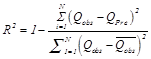(6)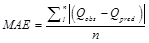(7)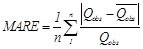(8)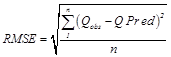(10)

Where Qobs is corresponds the steam flow observed value, Qpre is corresponds the predicted flow rate value, andis the average value of flow rate. MSE is mean square error and CE is coefficient of efficiency and n is number of data point used and n is number of observed data or sample of size.

The parameters used to evaluate and the validation model and the best conjunction wavelet transform with neural networks or neuro fuzzy predict are: The mean square error (MSE), defined by (Equation 5) and linear regression (equation 6) between actual the real data and simulated by the network.

4. Results and Discussions

The realiability of experimental setup is estabilished by comparing the performance of ANN and wavelet hybrid models of 588 month and 49 years. Due to the temporal serie of hydrological process its recomended to use 45 yers for first part for training equivalente of 80 percent than, the rest 4 yers for verificatior or test equivalente 20 percent of time series by figure 6.

Table 2 and figure 8 below shows training and test results of forecasting of flow in pungwe river basin bybusing WANFIS end WANN model with corresponding the Root-Mean-Squere (RMSE), Degree of correlation (R2) and Nash-Sutcliffe of efficiency (NSE) and coeficient of correlation (R).

Table 2. Performance results of the WANN and WANFIS model with diferent situation.

 Decomposition Training Testing WANN WANFIS WANN WANFIS WANN WANFIS R2 Sym3,3 Db3,4 0.8282 0.9061 0.6894 0.8706 RMSE Sym3,2 Db3,4 190.1096 139.57 179,60 123.68 NSE Sym3,2 Db3,4 0,843 0.861 0.4682 0.5261 R2 Coif1,3 Db5,3 0.6150 0.729 0.5382 0.5454 RMSE Coif1,3 Db5,3 199.42 178.83 172.57 164.212 NSE Coif1,3 Db5,3 0.8047 0.79 0.7813 -0.421

The value of degree correlation(R2) in WANFIS in Db3,4 is higher than WANN where in general the model shoul be greater than 0.5 and is considered as an acetable macth to the real system. Other situation using Nash-Sutclifficient of coeffient of efficiency I can see is varies between 0.46 to 0.843 which it mean the model efficient corresponds a perfect prediction.

Table 3 shows training and test results of forecasting of flow in pungwe river basin bybusing ANFIS end ANN model with corresponding the Root-Mean-Squere(RMSE), Degree of correlation(R2), Nash-Sutcliffe of efficiency(NSE) and coeficient of correlation(R), Coefficient of Correlation(CE) and Mean Absolute Error(MAE).

Table 3. Performance results of the ANN and ANFIS model with diferent situation.

 Time Training Testing ANN ANFIS ANN ANFIS ANN ANFIS R2 2,5 2,5 0.8710 0.978 0.7621 0.982 RMSE 2,5 2,5 128.61 185.43 105.06 146.06 NSE 2,5 2,5 0.9293 0.7813 0.8667 0.5831 MAE 2,5 2,5 134.3 107.07 122.05 97.45 CE 2,5 2,5 0.6770 0.872 0.567 0.856 R2 2,9 2,9 0.9326 0.971 0.9254 0.976 RMSE 2,9 2,9 115.64 132.76 85.4510 115.67 NSE 2,9 2,9 0.9109 0.8021 0.6420 0.7081 MAE 2,9 2,9 118.85 197.14 97.020 154.3 CE 2,9 2,9 0.9016 0.8902 0.8632 0.8871

Table 3 shows the performance  and summarize the values of ANFIS and ANN models for difernt layers, and we verify that the result are litle similar. The Root-Mean-Squere(RMSE) for both model was good betwen the 85.45 to 128.61 m3/s. Based on the results of table 2 and table 3, it was noticed that the number of decomposition levels had considerable impact on the results. Since the random parts of original time series were mainly in the first resolution level, obviously the prediction errors were also mainly in the first resolution level.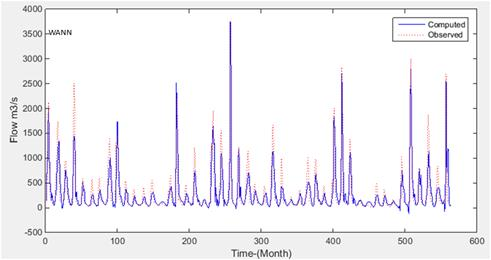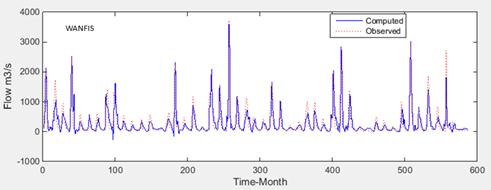Figure 6. Time series between obserced and computed WANN and WANFIS.

The figure 8 represent the scattter plots of observed flow data and and simulation during the validation time. The different between the regration line and 45o are close or de regretion value for all situation is aproximatley 1 and give clear that this data are corelected and it can be useful to built hydropower plant.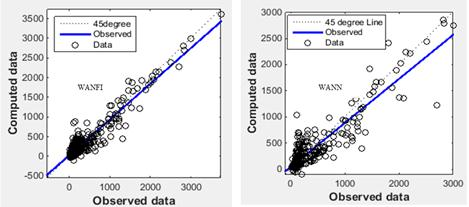Figure 8. Regression of WAFIS and WANN model.

5. Conclusions

The dataset used in this modelling experiment was a time series of average monthly river discharge during the period 1960–1994, which was collect from the Ara centro gauging hydrological station in the pungwe river basin Manica, and  this study applied time series models for different matlab program like ANN,WANN, ANFIS, WANFIS at Pungwe basin station  in Manica, Mozambique including the statistics tools ,RMSE, MAE, R2, were adopted to evaluate models performance. It was found that for almost all lead times WANN and WAFIS model has given better and consistent results compared to conventional ANN and ANFIS model. Also, the effect of decomposition level on WANN models efficiency was studied.

It was observed through this paper that the hybrid model is really have high efficiency because it possessed a high value linear regression 0.9548 combined with a good values of  Nash-Sutcliffe of efficiency(NSE) between 0.46 to 0.86.

Regards the flow prediction I can conclude that the flow presented in this river has a strong correlation and is good site to build a Small hydro power plant in Manica, than the climatic changes is not creat any problem about water to generate power.

Acknowledgement

The authors would like to thank the Department of Water Resources at Lund University and the Chemical department in Eduardo Mondlane University for help to carry out this beautifull Project.

References

1. EDM ( Electricidad de Mocambique), 2015. Stastitical Summary. www.edm.co.moz.
2. IEA, 2009. IEA Energy Statistics - Energy Balances for Mozambique. Available at: http://www.iea.org/stats/balancetable.aspAccessed May, 2015].
3. Cuamba B.C., Uthui R. Chenene M.L. et al.(unpubl.) Identification of areas with likely good wind regimes for energy applications in Mozambique. Eduardo Mondlane University, Maputo
4. Santos, C. A. G., Silva, G. B. L., (2014) Daily streamflow forecasting using a wavelet transform and artificial neural network hybrid models. Journal des Sciences Hydrologiques, 59 (2) 312–324.
5. Solgi, A., Radmanesh,F., Zarei,H., Nourani, V., (2014), Hybrid Models Performance Assessment to Predict Flow of Gamasyab River International journal of Advanced Biological and Biomedical ResearchVolume 2, Issue 5, 2014: 1837-1846.
6. O. Kisi.,(2008). "Stream flow forecasting using neuro-wavelet technique,"Hydrological Processes, vol. 22, no. 20, 4142–4152.
7. FUNAE (Fundo de Energia), 2015. Annual Report. www.funae.co.moz.
8. V. Nourani, M. T. Alami, and M. H. Aminfar, (2009), "A combined neural-wavelet model for prediction of Ligvanchai watershed precipitation," Engineering Applications of Artificial Intelligence, Vol. 22, no. 3, 466–472.
9. Nourani V, Hosseini Baghanam A, Adamowski J, Gebremichael M, (2013), Using self-organizingmaps and wavelet transforms for space–time preprocessing of satellite precipitation and runoff data in neural network based rainfall–runoff modeling. J Hydrol 476:228–243.
10. Sreekanth, P., Geethanjali, D.N., Sreedevi, P.D., Ahmed, S., Kumar, N.R., Jayanthi, P.D.K., (2009),Forecasting groundwater level using artificial neural networks. Current Science 96 (7), 933–939.
11. Mohammadi, K., (2008), Groundwater table estimation using MODFLOW and artificial neural networks. Water Science and Technology Library 68 (2), 127– 138.
12. Nourani,V., Hosseini, A., Adamowski, J., Kisi,O, (2014), Applications of hybrid wavelet–Artificial Intelligence models in hydrology: A review, Journal of Hydrology 514 , 358–377.
13. Partal,T., Kisi, Ö. (2007), Wavelet and neuro-fuzzy conjunction model for precipitation forecasting. Jornal of Hydrology, 342,199-212.
14. Krishna, B.; Satyaji Rao, Y. R., Naya, P.C.(2011) Times Series Modeling of River Flow Using Wavelet Neural Networks, Journal of water resource and protection, 3, 50-59.
15. Nayak P.C., Venkatesh B., Krishna,B., Sharad, K J., (2013) Rainfall-runoff modeling using conceptual, data driven, and wavelet based computing approach. Journal of Hydrology 493 57–67.
16. Rezaeianzadeh, M., Tabari, H, Yazdi, A. A., Isik, S., and Kalin, L. (2014)."Flood flow forecasting using ANN, ANFIS and regression models." Neural Computing and Applications, Vol. 25, Issue 1, pp. 25-37, DOI: 10.1007/s00521-013-1443-6.
17. Nejad, F. H., and Nourani, V. (2012). "Elevation of wavelet denoising performance via an ANN-based streamflow forecasting model." International Journal of Computer Science and Management Research, Vol. 1, Issue 4, pp. 764-770.
18. Kisi, O. (2006). "Streamflow forecasting using different artificial neural network algorithms." Journal of Hydrologic Engineering, Vol. 12, Issue 5, pp. 532-539, DOI: 10.1061/(ASCE)1084-0699(2007)12:5(532).
19. Kim, T.W., Valdes, J.B., 2003.Nonlinear model for drought forecasting based on a conjunction of wavelet transforms and neural networks. Journal of Hydrologic Engineering 6, 319–328.
20. Nourani, V., Kisi, Ö., Komasi, M., 2011.Two hybrid artificial intelligence approaches for modeling rainfall-runoff process. Journal of Hydrology 402, 41–59.
21. Nourani, V., Baghanam, A.H., Adamowski, J., Gebremichael, M., 2013. Using selforganizing maps and wavelet transforms for space–time pre-processing of satellite precipitation and runoff data in neural network based rainfall-runoff modeling. Journal of Hydrology 476, 228–243.
22. Dibike, Y.B., Solomatine, D.P., 2001. River flow forecasting using artificial neural networks. Physics and Chemistry of the Earth, Part B: Hydrology, Oceans and Atmosphere 26, 1–7.
23. Badrzadeh, H., Sarukkalige R., Jayawardena,A.W., 2013.Impact of multi-resolution analysis of artificial intelligence models inputs on multi-step ahead river flow forecasting.Journal of Hydrology 507,75–85.
24. Jang, J.S., 1993. ANFIS: adaptive-network-based fuzzy inference system. IEEE Transactions on Systems, Man and Cybernetics 23, 665.
25. Chiu, S., 1994. Fuzzy model identification based on cluster estimation. Journal of Intelligent & Fuzzy Systems 2.
26. Uamusse, M., Persson, K. and Tsamba, A. (2014) Gasification of Cashew Nut Shell Using Gasifier Stovein Mozambique. Journal of Power and Energy Engineering, 2, 11-18. doi: 10.4236/jpee.2014.27002.

 Contents 1. 2. 3. 3.1. 3.2. 3.3. 3.4. 4. 5.
Article ToolsAbstractPDF(569K)